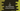# C program to add two distances in feet and inches using structure## C program to add two distances in feet and inches using structure:

In this post, we will write one C program that can add two distances using a structure. This program will take the distance values in feet and inches from the user and add them. The structure we will create can hold feet and inches values. You can make the code more readable by using a structure.

Let’s write down the program.

### C program:

Below is the complete program:

``````#include <stdio.h>

struct d
{
int feet;
int inch;
};

struct d add(struct d first, struct d second)
{
struct d r;

r.feet = first.feet + second.feet + (first.inch + second.inch)/ 12;
r.inch = (first.inch + second.inch) % 12;

return r;
}

int main(void)
{
struct d firstDistance, secondDistance;
printf("Enter the value of feet and inches for the first distance: ");
scanf("%d%d", &firstDistance.feet, &firstDistance.inch);

printf("Enter the value of feet and inches for the second distance: ");
scanf("%d%d", &secondDistance.feet, &secondDistance.inch);

struct d resultDistance = add(firstDistance, secondDistance);

printf("Final distance feet: %d, and inches: %d\n", resultDistance.feet, resultDistance.inch);

return 0;
}``````

### Explanation:

Here,

• struct d is the structure to hold both feet and inch values.
• add method is used to add the values of two structures of type d. This method returns one structure of type d.
• We have initialized one new structure r in this method. This will hold the final result. The feet is the sum of feet values of both structures and the feet value of sum of the inches. Since 12 inches is equal to one feet, we are dividing the sum of inches by 12.
• Similarly, the inches is calculated as sum of inches values modulo 12.
• The main method is called first. It initialized two structure d variables firstDistance and secondDistance to hold the user input distance values. It takes the values as input from the user and stores them in firstDistance and secondDistance. Then it passes these values to add to find the sum. This result is stored in another structure d variable resultDistance.
• The final line prints the feet and inch values of this result variable.

### Sample output:

If you run this program, it will print output as like below:

``````Enter the value of feet and inches for the first distance: 20 10
Enter the value of feet and inches for the second distance: 30 10
Final distance feet: 51, and inches: 8``````